# 4. Below are production functions that turn capital (K) and labor (L) into output. For each...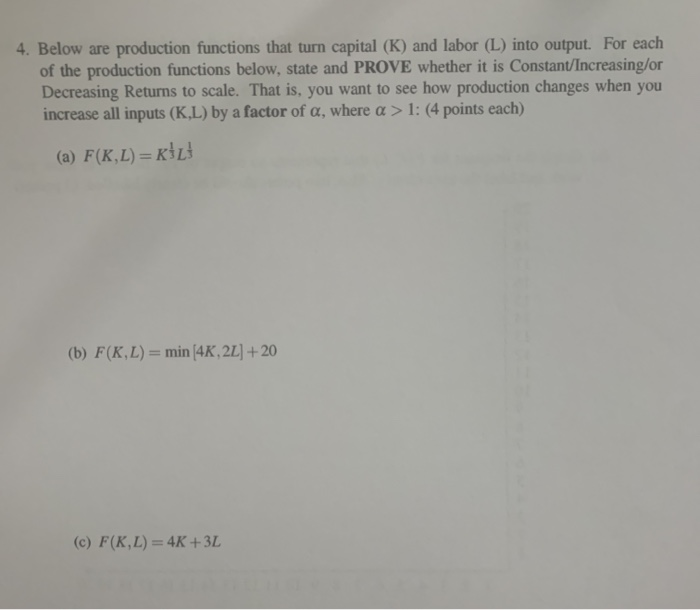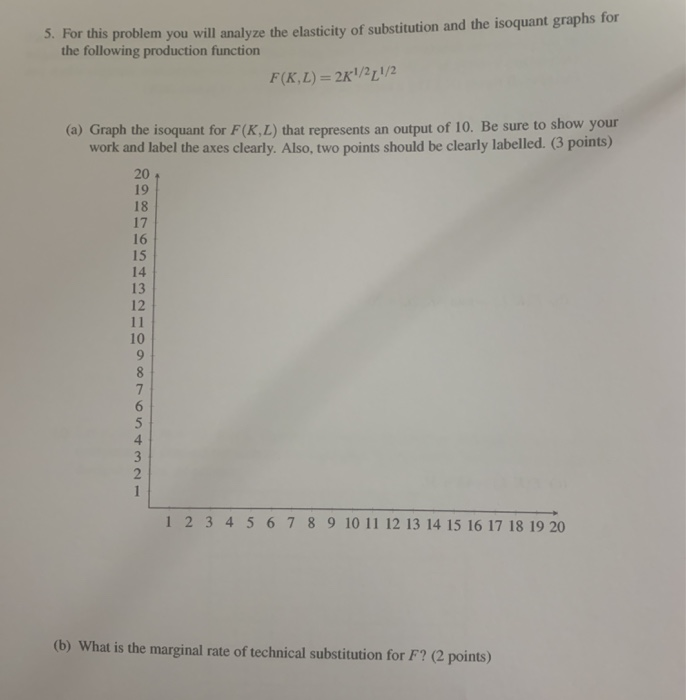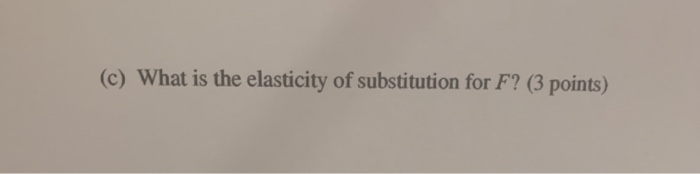4. Below are production functions that turn capital (K) and labor (L) into output. For each of the production functions below, state and PROVE whether it is Constant/Increasing/or Decreasing Returns to scale. That is, you want to see how production changes when you increase all inputs (K.L) by a factor of a, where a > 1: (4 points each) (a) F(K,L) =KİL (b) F(K,L) = min 4K, 2L] + 20 (c) F(K,L) = 4K +3L
5. For this problem you will analyze the elasticity of substitution and the isoquant graphs for the following production function F(KL) = 2K1/2 1/2 (a) Graph the isoquant for F(K.L) that represents an output of 10. Be sure to show your work and label the axes clearly. Also, two points should be clearly labelled. pomis) 18 1 2 3 4 5 6 7 8 9 10 11 12 13 14 15 16 17 18 19 20 (b) What is the marginal rate of technical substitution for F? (2 points)
(c) What is the elasticity of substitution for F? (3 points)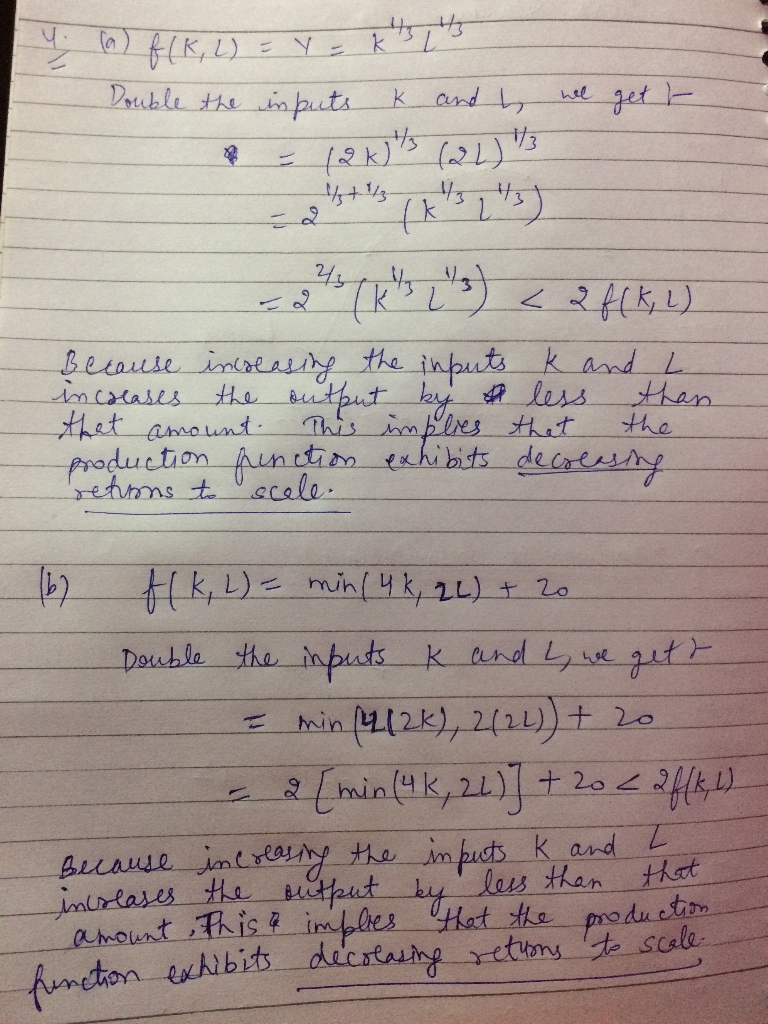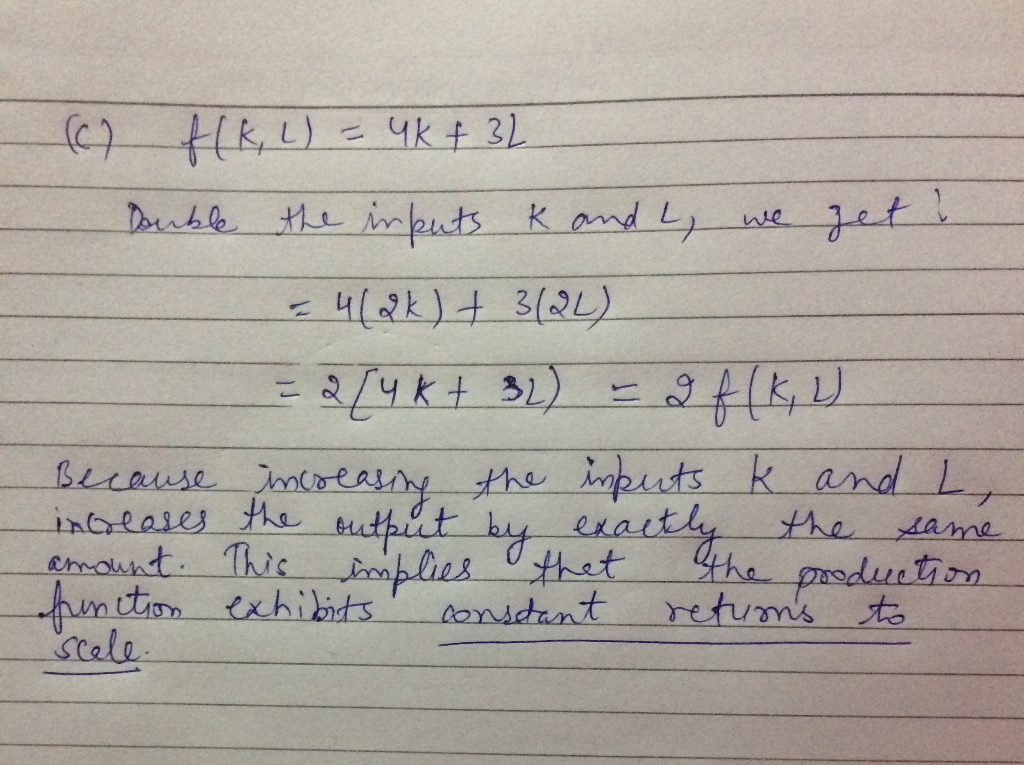#### Earn Coin

Coins can be redeemed for fabulous gifts.

Similar Homework Help Questions
• ### 1. Below are production functions that turn capital (K) and labor (L) into output. For each...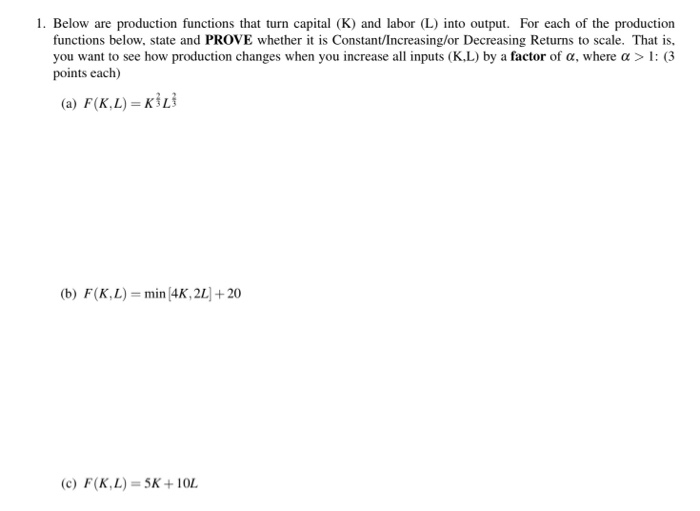1. Below are production functions that turn capital (K) and labor (L) into output. For each of the production functions below, state and PROVE whether it is Constant/Increasing/or Decreasing Returns to scale. That is, you want to see how production changes when you increase all inputs (KL) by a factor of a, where a > 1: (3 points each) (a) F(K.L) = (b) F(KL)= min (4K, 2L + 20 (c) F(K,L) = 5K+ 10L

• ### 1. Below are production functions that turn capital (K) and labor (L) into output. For each...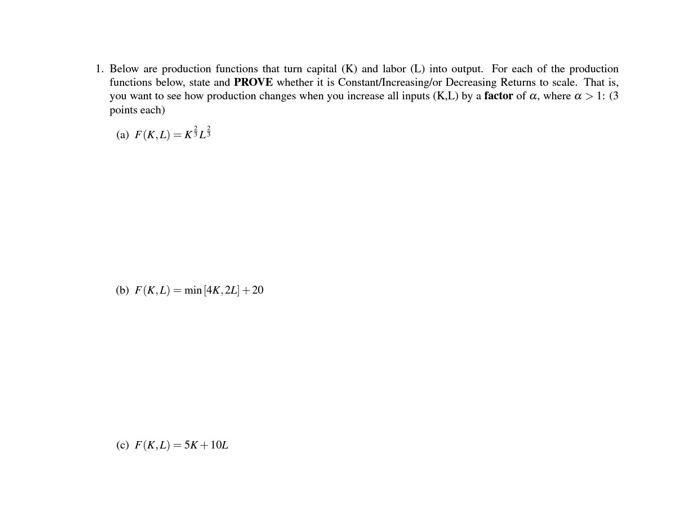1. Below are production functions that turn capital (K) and labor (L) into output. For each of the production functions below, state and PROVE whether it is Constant/Increasing/or Decreasing Returns to scale. That is, you want to see how production changes when you increase all inputs (K.L) by a factor of a, where a > 1: (3 points each) (a) F(KL)=KL (b) F(K,L) = min (4K, 22] + 20 (c) F(K,L) = 5K+10L

• ### 1. Below are production functions that turn capital (K) and labor (L) into output. For cach of th...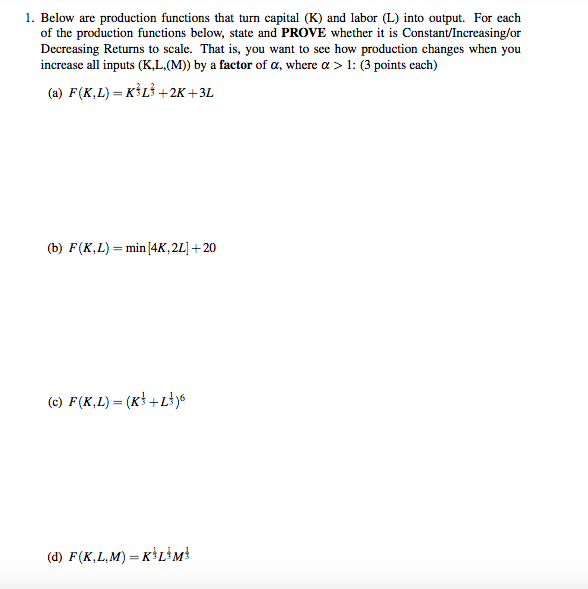1. Below are production functions that turn capital (K) and labor (L) into output. For cach of the production functions below, state and PROVE whether it is Constant/Increasing/or Decreasing Returns to scale. That is, you want to see how production changes when you increase all inputs (K,L, (M)) by a factor of a, where a > 1: (3 points each) (a) F(K,L)-KİLİ+2K +3L (b) F(K, L)=min/4K, 2L1+20 (d) F(K,L,M) KL3M 1. Below are production functions that turn capital (K) and...

• ### 5. For this problem you will analyze the elasticity of substitution and the isoquant graphs for...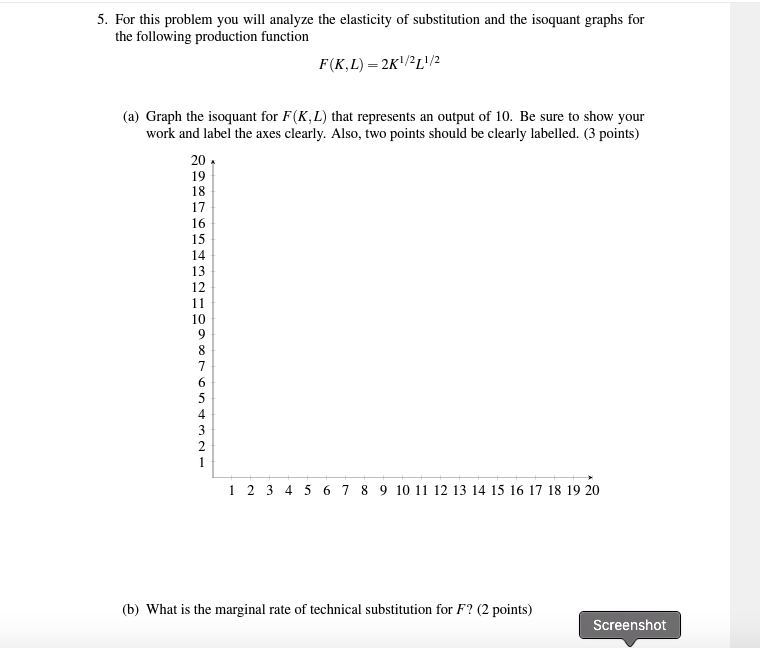5. For this problem you will analyze the elasticity of substitution and the isoquant graphs for the following production function F(K.L) = 2K1/2 1/2 (a) Graph the isoquant for F(K,L) that represents an output of 10. Be sure to show your work and label the axes clearly. Also, two points should be clearly labelled. (3 points) 1 2 3 4 5 6 7 8 9 10 11 12 13 14 15 16 17 18 19 20 (b) What is the...

• ### Below are production functions that turn capital (K) and labor (L) into output. For each of...

Below are production functions that turn capital (K) and labor (L) into output. For each of the production functions below, state and PROVE whether it is Constant/Increasing/or Decreasing Returns to scale. That is, you want to see how production changes when you increase all inputs (K,L,(M)) by a factor of α, where α > 1: (4 points each) a) F(K,L) = K^1/3*L^1/3+2K+3 b) F(K,L) = sqr(K^3+L^3) c) F(K,L) = (K^2/4+L^2/4)^2 d) F(K,L,M) = min(K,L)*M

• ### 2. For this problem you will analyze the elasticity of substitution and the isoquant graphs for...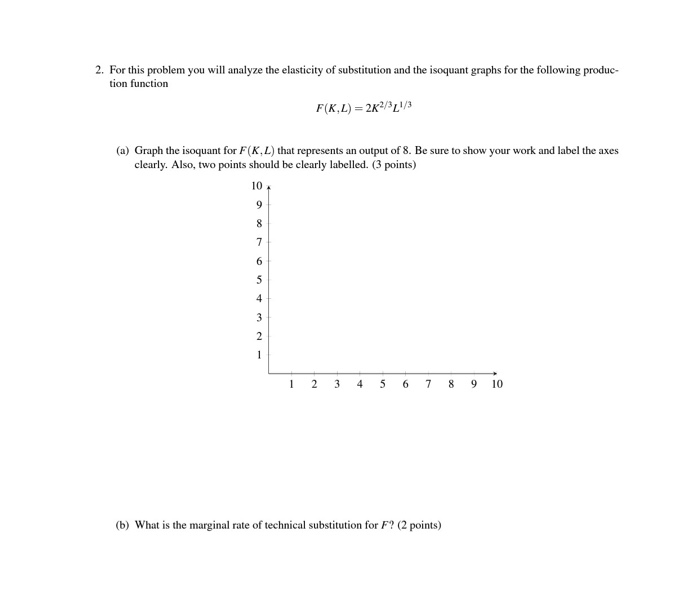2. For this problem you will analyze the elasticity of substitution and the isoquant graphs for the following produc- tion function F(K.L) 2K2/L/3 (a) Graph the isoquant for F(K,L) that represents an output of 8. Be sure to show your work and label the axes clearly. Also, two points should be clearly labelled. (3 points) 10 9 7 6 5 4 3 2 6 7 89 10 2 3 4 5 (b) What is the marginal rate of technical substitution...

• ### 3. For each of the following production functions, graph a typical isoquant and determine whether the marginal rate of...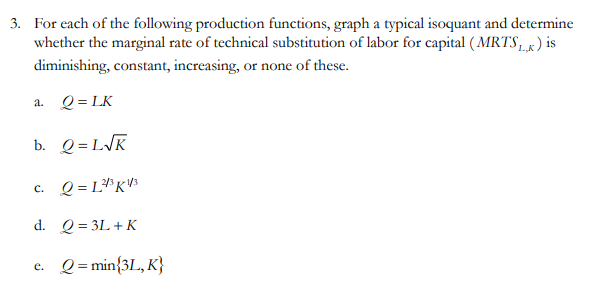3. For each of the following production functions, graph a typical isoquant and determine whether the marginal rate of technical substitution of labor for capital (MRTS ) is diminishing, constant, increasing, or none of these. a. Q=LK b. Q=LVK c. Q=L*K13 d. Q = 3L +K e. Q = min{3L, K} Show transcribed image text 3. For each of the following production functions, graph a typical isoquant and determine whether the marginal rate of technical substitution of labor for capital...

• ### Given production function Q-fCK, L)-8KL +、/L where K is capital and L is labor. b. Define...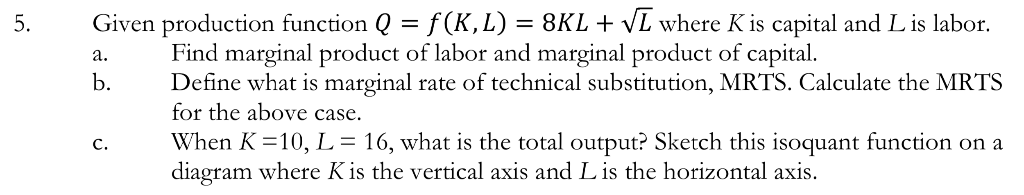Given production function Q-fCK, L)-8KL +、/L where K is capital and L is labor. b. Define what is marginal rate of technical substitution, MRTS. Calculate the MRTS c. When K = 10, L-16, what is the total output? Sketch this isoquant function on a Find marginal product of labor and marginal product of capital. for the above case. diagram where Kis the vertical axis and L is the horizontal axis

• ### 1. [30 POINTS] Consider the production function y=f(L,K) = 4/1/2K1/4 where L is labor and K...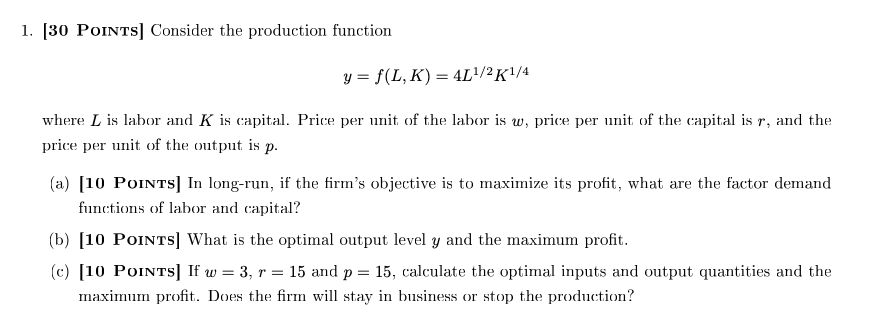1. [30 POINTS] Consider the production function y=f(L,K) = 4/1/2K1/4 where L is labor and K is capital. Price per unit of the labor is w, price per unit of the capital is r, and the price per unit of the output is p. (a) (10 POINTS] In long-run, if the firm's objective is to maximize its profit, what are the factor demand functions of labor and capital? (b) (10 Points) What is the optimal output level y and the...

• ### 5. Suppose that an economy has a fixed amount L* of homogeneous labour, and a fixed amount K*of h...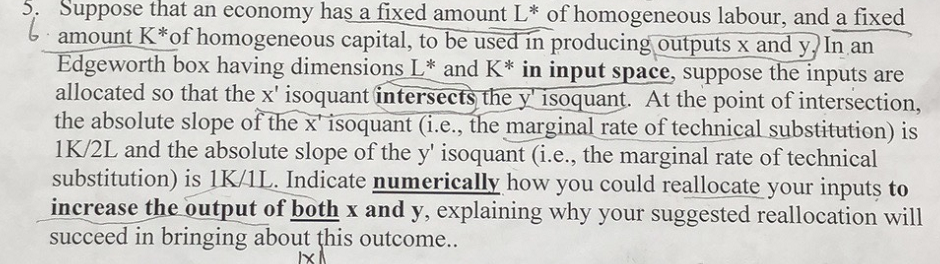5. Suppose that an economy has a fixed amount L* of homogeneous labour, and a fixed amount K*of homogeneous capital, to be used in producing outputs x and y) In an Edgeworth box having dimensions L and K* in input space, suppose the inputs are the absolute slope of the x isoquant (i.e., the marginal rate of technical substitution) is 1K/2L and the absolute slope of the y' isoquant (i.e., the marginal rate of technical substitution) İs l K/L indicate...